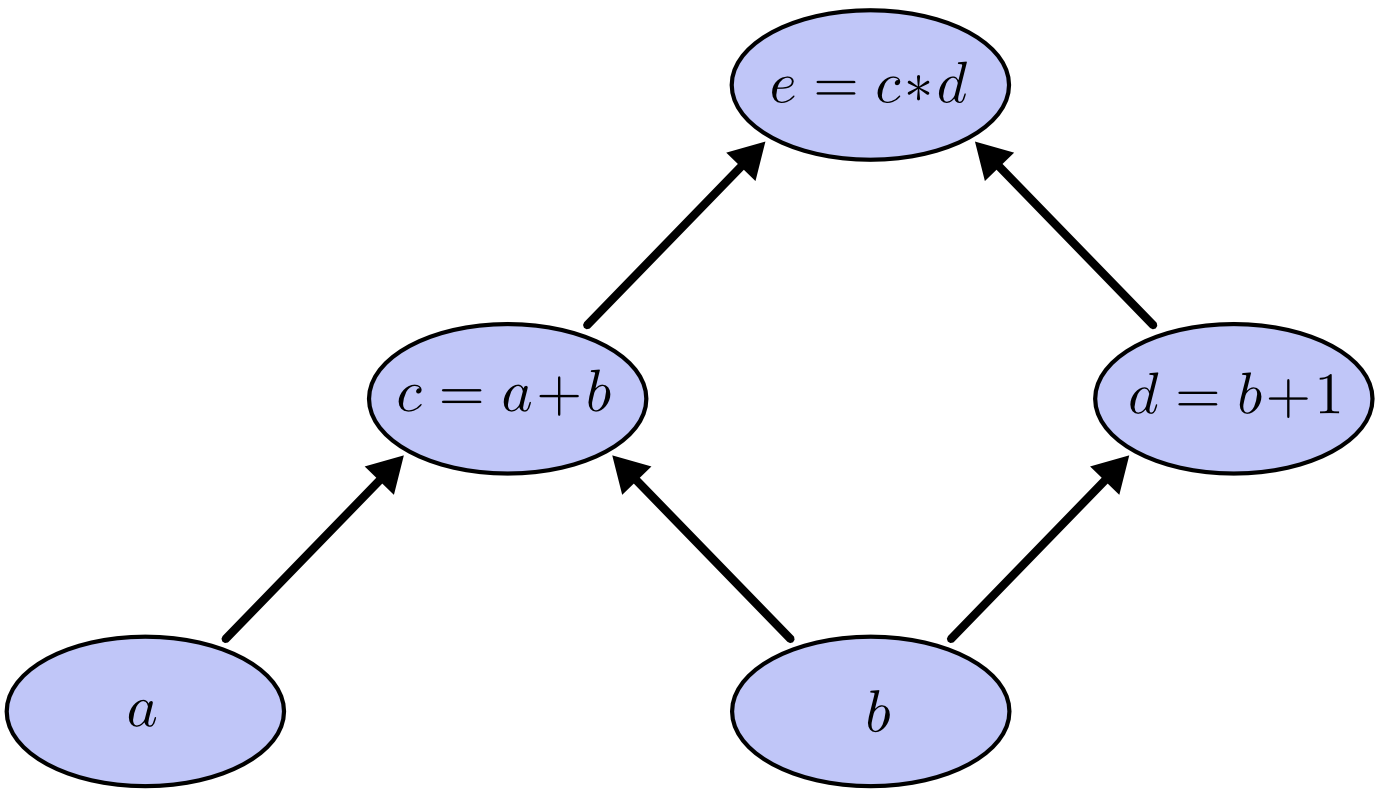# Autograd (and some fun stuffs) came to noe

Thursday, January 30, 2020

Implementing automatic gradient computation, or simply autograd, is pretty challenging to me. Especially when the language that I use (and love) does not really have the tools that I really need, e.g., the multi-dimensional array. Thus, I ended up implementing one. It pretty much functional although the are still many aspect to tune. With noe providing tensor representation, I attempted to implement an automated gradient computation mechanism. Something like what other deep learning frameworks do to perform neural network parameter optimization.

## Where did I start off

Obviously I had no background knowledge to do this. I simply wanted to do the magic other framework do. So I googled a bit and encountered some nice introductory materials.

• A video lecture by Matthew James Johnson, one of the guy behind HIPS autograd framework. The lecture was good and gave me a bit understanding of what happens under the hood of the autograd. It turned out he also made the minimum version (autodidact) of HIPS autograd that seems easier for anyone who wants to replicate or reimplement in other programming languages. But the fancy dynamic language features in python he used were too much to handle for me who use pascal.
• A github repo by Utku Evci. What he has implemented was very minimal (so it is good for me) and does not really require fancy language-specific features. Just plain object-oriented approaches with some operator overloading that you can find in almost any languages. The API looks like pytorch's, which I am familiar with. So I chose this repo as my starting point.

It turned out that autograds out there are implemented based on a computational graph. Christoper Olah explained it very well in his blog. Consider following expressions:

$$c = a+b$$ $$d = b+1$$ $$e = c*d$$

All these operations including the variables are represented as nodes. Visually (and blatantly taken from Olah's blog) the resulted graph will look like this:## Pascal implementation with noe

My implementation seems close to pytorch, i.e., the tensor that holds the data is wrapped inside a variable. The variable holds the gradient information and other required information for building and operating the computational graph.

uses
noe.core,
noe.math,
noe.op.base;

var
A: TTensor;
X, Y: TVariable;

begin
{ rank-1 noe tensor containing values 0...9 }
A := Range(10);

{ Tensor A is then wrapped inside a TVariable }
X := TVariable.Create(A);

{ We set RequiresGrad to true as we want to compute
the gradient of a function w.r.t. X }
...


Then we determine Y as the function of X (for example, $y = 2x^2-2$). Subsequently Y.Backpropagate triggers the computation of the gradient for all nodes in the graph.

    ...

{ y = 2x^2 - 2 }
Y := 2 * X * X - 2;
Y.Backpropagate;

Writeln('X ='); PrintTensor(X.Data); Writeln;
Writeln('Y ='); PrintTensor(Y.Data); Writeln;

end.



The output:

X =
[0.00, 1.00, 2.00, 3.00, 4.00, 5.00, 6.00, 7.00, 8.00, 9.00]

Y =
[-2.00, 0.00, 6.00, 16.00, 30.00, 48.00, 70.00, 96.00, 126.00, 160.00]

dY/dX =
[0.00, 4.00, 8.00, 12.00, 16.00, 20.00, 24.00, 28.00, 32.00, 36.00]


It is quite unsatisfying if I cannot verify visually. So I decided to make graphical visual display possible.

## Plotting the data

At this moment, relying the LCL or even creating a plotting system will surely consume lots of time. Moreover, I want to be able to show plot regardless the project being GUI or console application. What came to my mind first was to make the interface to an existing plotting program. In this regard, I use GNU plot. The detailed API for plotting will be on a separated post. Essentially, the wrapper generates the script which is then executed by GNU plot program. This is the simplest and the fastest, I think. Why not just use GNU plot directly? Because I don't want the user to hop programming or scripting language just to display the visual. Moreover, piping the tensor data as the result of computation to GNU plot is a nontrivial task, especially when the data is big.

First, add the required unit and declare the required variable for plotting.

uses
...
noe.plot.gnuplot;

var
...
plot1, plot2: TPlot;

begin
...
...
...

GNUPlotInit('gnuplot');
figure := TFigure.Create;
figure.Title := 'y = 2x^2 - 2';

plot1 := TPlot.Create;
plot1.PlotType := ptLines;
plot1.Title := 'y';
plot1.SetDataPoints(Y.Data); // y

plot2 := TPlot.Create;
plot2.PlotType := ptLines;
plot2.Title := 'dy/dx';

figure.Show;

end.


Having ensured the gnuplot executable is already in the system search path, the output will be like this.We can also use trigonometric functions.

    { rank-1 noe tensor containing linearly spaced values from -5 to 5
with step size of 0.1 }
A := Range(-5, 5, 0.1);

X := TVariable.Create(A);

Y := Tanh(X);
Y.Backpropagate;


The we display the result.You may see the complete functions in noe github repository here. Note that at this moment, noe only support computation of first-order derivative. The implementation of higher order derivative is in my plan. But not now, as it is not that crucial.

Having the autograd implemented, we can now move toward the development of neural networks. But, again, that will be in the separated post. Cheers.

#machine learning#development